If you have a related question, please click the "Ask a related question" button in the top right corner. The newly created question will be automatically linked to this question.

# THS4531: The design bandwidth of the differential circuit does not match the bandwidth value obtained from simulation.

Part Number: THS4531
Other Parts Discussed in Thread: TINA-TI, THP210

Hi,

Good day.

I want to use THS4531 to design a multiple feedback (MFB) low-pass filter (LPF) circuit, in order to amplify the differential signal.

The MFB LPF circuit is shown in the following figure:Now, I have encountered some problems:

According to theoretical calculations, the transfer function of the filter isand the cutoff frequency fc isQ is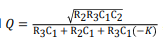Here are the parameters in THS4531 Evaluation Module:https://www.ti.com/tool/THS4531DGKEVM

R1 = 2k ohms;

R2 = 2k ohms;

R3 = 255 ohms;

C2 = 200   pF;

C1 = 9100   pF;

Substitute the above parameters into the formula calculation to obtain the cutoff frequency fc:

fc = 58.986k Hz

However, I build a simulation model in Tina-Ti, as shown in the following figure: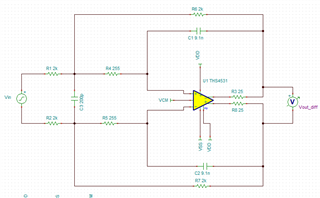Subsequently, the AC transmission characteristics of the circuit were analyzed, and the results are shown in the figure: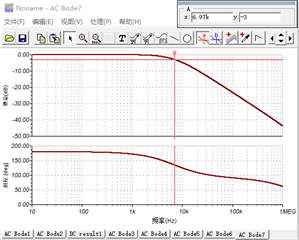At -3db, the frequency is 6.97k which is not equal to 58.986k Hz.

My question is why is this happening? Why is the effective bandwidth smaller than what my calculations tell me they should be. I can't find out what I did wrong with my calculations, and I am not sure if there are any other equations to consider.

Thank you and stay safe.

Regards,

Lewis

• Hi Lewis,

one mistake is that you should double the capacitance of C2 when moving from a single ended to a differential design.

Kai

• And your filter component values are wrong: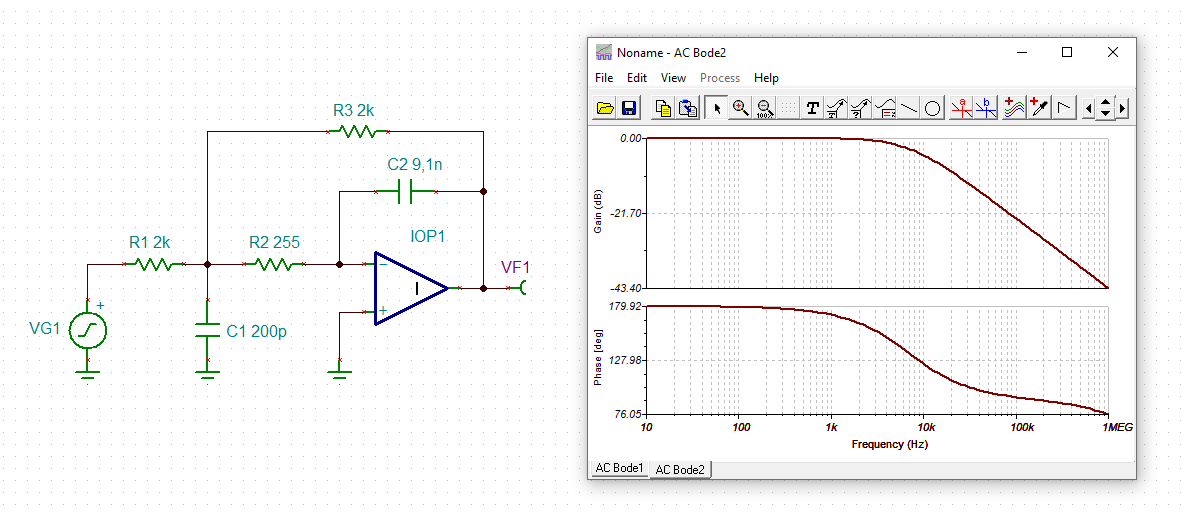Kai

• Kai, you actually need to 1/2 the single ended value cap value at the inputs when making it a diff design

Let me assume Lewis you were trying to get a 60kHz, Q=0.707. The legacy design flows peak the noise gain unnecessarily, here is a better set of RC,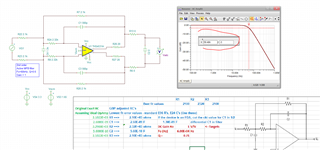And this file,

THS4531a 60kHz MFB.TSC

• And something I always like to look at in active filters is the LG over the frequency span, that is here for the values I sent - these relatively high values of LG at peak NG indicate the GBP for the target filter is quite high, you could also look at the THP210 FDA if you cared a lot about DC precision.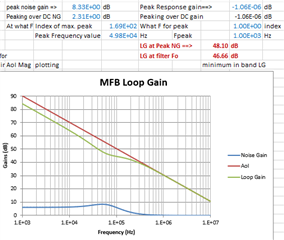• Kai, you actually need to 1/2 the single ended value cap value at the inputs when making it a diff design

Yes, of course. Thanks for the correction.

Kai

• Hi Kai，

Indeed, I did forget to 1/2 the single ended value cap value at the inputs....

Lewis

• Hi Michael,

After reading your answer, I realized that I had overlooked an important question —— I confused the concept between filter characteristic frequency f0 and cutoff frequency fc.

When designing MFB-LPF's parameters, the value of Q was not taken into account, which resulted in the characteristic frequency f0 calculated by the formula not being equal to the cutoff frequency fc obtained through simulation.  That is my fault...